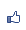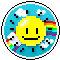唐老鴨之家 市長：燢 　副市長： 加入本城市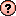｜推薦本城市｜加入我的最愛｜訂閱最新文章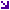本城市首頁討論區精華區投票區影像館推薦連結公告區訪客簿市政中心 (0)繪製中華民國國旗的VBA程式(原創) 瀏覽1,075｜回應0｜推薦1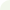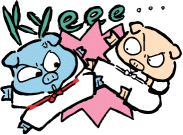燢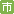等級：6 留言｜加入好友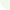文章推薦人 (1)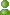龍女CHANG, HSIU-FEN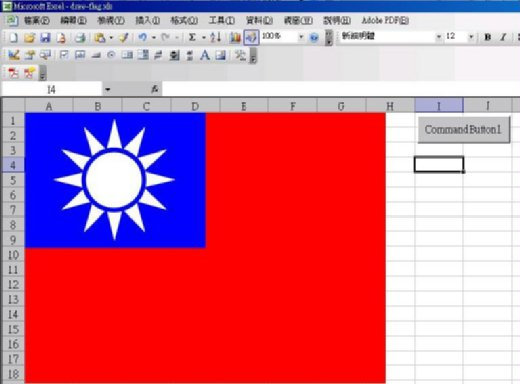Private Sub CommandButton1_Click()
Rem draw the red block
Selection.ShapeRange.Fill.ForeColor.SchemeColor = 2
Selection.ShapeRange.Line.ForeColor.SchemeColor = 2

Rem draw the blue block
Selection.ShapeRange.Fill.ForeColor.SchemeColor = 4
Selection.ShapeRange.Line.ForeColor.SchemeColor = 4

Rem draw the 12 light
r1 = 400 / 6
r2 = 400 / 12
ww = 400 / 4
hh = 300 / 4
For i = 1 To 12
theta = i * 6.283 / 12
X1 = ww + r2 * Cos(theta + 6.283 / 24)
Y1 = hh + r2 * Sin(theta + 6.283 / 24)
X2 = ww + r1 * Cos(theta + 6.283 / 12)
Y2 = hh + r1 * Sin(theta + 6.283 / 12)
X3 = ww + r2 * Cos(theta + 6.283 / 8)
Y3 = hh + r2 * Sin(theta + 6.283 / 8)
With ActiveSheet.Shapes.BuildFreeform(msoEditingAuto, X1, Y1)
.ConvertToShape.Select
End With
Selection.ShapeRange.Line.ForeColor.SchemeColor = 9
Selection.ShapeRange.Fill.ForeColor.SchemeColor = 9
Next i

Rem draw the circle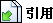引用網址：https://city.udn.com/forum/trackback.jsp?no=58536&aid=4752757